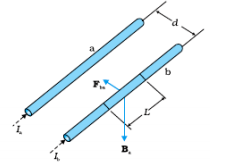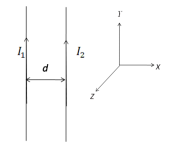Two long straight parallel conductors carry steady current and ${I}_{2}$ separated by a distance d. If the currents are flowing in the same direction,show how the magnetic field set up in one produces an attractive force on the other. Obtain the expression for this force. Hence define one ampere.                 (3)$\left(1}{2}\right)$

As shown in figure,the direction of force on conductor b is attractive  $\left(1}{2}\right)$   [Alternatively:

$\stackrel{\to }{B}$ at a point on wire 2, is long -$\stackrel{^}{k}$

$\therefore$$\stackrel{\to }{F},$on wire 2 , due to the $\stackrel{\to }{B}$,is a long -$\stackrel{^}{l}$,i.e.

towards wire1. Hence the force is attractive.Magnetic field,due to current in conductor a,

${B}_{1}=\frac{{u}_{0}{I}_{1}}{2\pi d}$                                           $\left(1}{2}\right)$

The magnitude of force on a length L of conductor b,

${F}_{2}={I}_{2}L{B}_{1}$                                                       $\left(1}{2}\right)$

${F}_{2}=\frac{{u}_{0}{I}_{1}{I}_{2}L}{2\pi d}$

One amphere is that steady current which, when maintained in each of the two very long, straight, parallel conductors, placed one meter apart in vacuum,would produce on each of these conductors a force equal to $2×{10}^{-7}$ newton per meter of their length.                                                                           (1)

Difficulty Level: